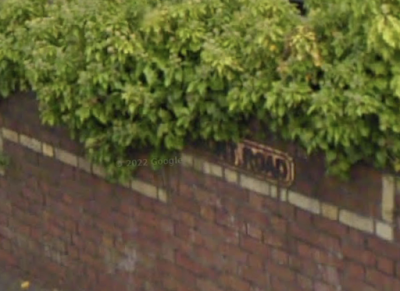## 2011-07-30

### Winding me up!

There are a couple of main jobs my polygon library has to do. One is making a set of overlapping polygons in to a set of simple non overlapping polygons. The other is working out which polygons are inside others so that we can do the basic Boolean maths on them.

The clip function turned out to be relatively simple, thankfully. And I also have a function to tidy up the polygons removing dead ends and unnecessary mid points on straight lines.

The problem is working out which polygons are inside others. My plan was simply to use the winding number logic, which as I said was simple! Basically, to find the winding number of a point, draw a line to infinity (I chose a line going left) and see what lines you cross. If they cross one way the winding number goes up, the other way it goes down. There are a few edge cases (sorry about the pun) where your line just touches another polygon but not crossing it, but correct use of greater than or equals in the right places and you avoid problems.

My plan was to find the winding number of each polygon. I.e. if it was clockwise the winding number of points immediately inside it, and if anti-clockwise the winding number of points immediately outside it. That is the winding number of points on your right as you walk around the polygon. That way I can do simple logic like union which means just keeping all polygons with winding number of 1 or intersection which means keeping all those with winding number of 2.

My plan was to take the left most point of each polygon (which I also use to work out if clockwise or anti-clockwise) and find the winding number of that point. Simples! or so I thought.

Of course I was immediately hit by a problem - which was pretty obvious. When working on the 3D models I am doing operations on adjacent slices to help find the top and bottom layers of things. This meant I was often working on identical polygons on top of each other. The clip logic already removed any segments that cancel each other out (i.e. clockwise on top of anti clockwise), but when two polygons are the same they were getting the same winding number (Doh!). The system needed to make an arbitrary decision and put one logically inside the other so giving one a bigger winding number.

My naive solution was to have a special case for where the X point I found was the same as I started with rather than on the left, and only count if it was on a polygon earlier in my list. This did work for the case of identical polygons as it meant one polygon was logically inside the other.

Sadly that was naive in the extreme. Such cases were just a special case of the more general problem where the point I am looking at is the same. If the polygons are in fact different, perhaps on the right, then clearly one is inside the other - you just cannot tell from the one point you are looking at.

So the plan now is to handle the case where the point is the same by following the polygons round until we either confirm it is the same all round (and then pick first in the list of polygons as a decider), or one branches off left or right. Thankfully the case where they go different directions is already catered for in the clip logic as the segments cancel out each other - so this means I can rely on the polygons going the same way (my link list only goes forward!). I have also removed points in the middle of straight lines. So I can just keep going until the points are different and then decide if this is a turn left or right of my original polygon.

I'll try the logic later, when I have done some real work.